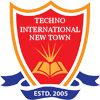#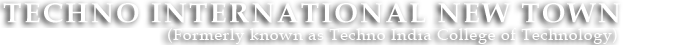+91 9674112076/2079
•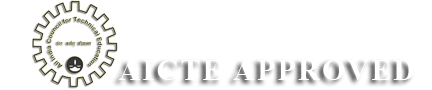••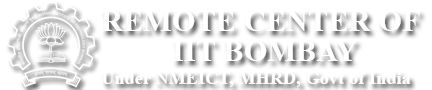•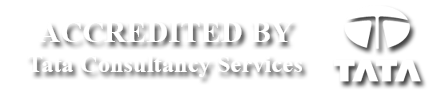# Signal System Lab

SIGNAL & SYSTEM LABORATORY

Code: EC 393
List of Experiments:
1. To study Z- transform of: a) Sinusoidal signals b) Step functions.
2. To compare Fourier and Laplace transformations of a signal.
3. To study convolution theorem in time and frequency domain.
4. To Study Signal Synthesis via sum of harmonics.
5. To study LPF &HPF, band pass and reject filters using RC circuits.
6. To demonstrate how analog signals are sampled and how different sampling rates affect the outputs.
7. To study sampling theorem for low pass signals and band pass signals .
8. To determine the components of: a) Square wave b) Clipped sine wave.